# Collision Theory in Equilibria

This is part of the HSC Chemistry syllabus under the topic of Static and Dynamic Equilibrium

### HSC Chemistry Syllabus

• Investigate the relationship between collision theory and reaction rate in order to analyse chemical equilibrium reactions (ACSCH070, ACSCH094

### How does collision theory help us better understand equilibrium systems?

This video will explore what collision theory is and how it relates to how we understand equilibrium systems

### What is Collision Theory?

Collision theory is a theory which states chemical reactions are the result of collisions between molecules or atoms.

It utilises our understanding of the particle model to explain that the rate of reaction depends on:

1. Rate of collision between molecules. This is the frequency at which molecules collide. Greater rate of collision leads to greater reaction rate.

2. Activation energy of the reaction. For a reaction to occur, the reactants must overcome a certain amount of activation energy. Even if the collision rate is high, if the particles don’t have enough energy reaction will still not occur.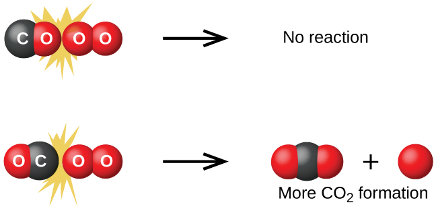Figure: Molecular orientation is an important factor of reaction rate as stated by collision theory. If the orientation of reactants is not correct (effective) during collision, reaction does not occur.

3. Molecular orientation of reactants. Rate of reaction can increase if reactants collide in the right orientation. Conversely, rate can decrease if the orientation of molecules is not favourable for the formation of product(s).

### What Affects Collision Rate?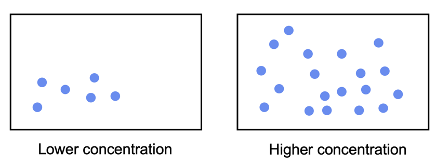Collision rate increases at a higher concentration

• Concentration of particles/molecules. A higher concentration means there are more particles moving about in a given volume, increasing the frequency at which particles collide.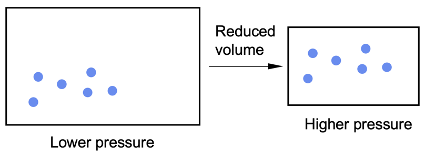Collision rate increases at a higher pressure for gases

• Pressure/volume. Changes in pressure and volume affect the collision rate between gaseous particles as they occupy the most volume. An increase in pressure (or reduction in volume) increases the rate of collision.
• Temperature determines the amount of heat energy in a system. A higher temperature (greater energy) means particles move about quicker at greater kinetic energy. This in turn increases the collision rate between them.

### What Affects Activation Energy?Catalyst reduces the activation energy

• Catalysts reduce the activation energy of a reaction, resulting in an increased rate.

### Molecular Energy Distribution

• In addition to increasing the collision rate, a higher temperature also allows more collisions to result in a chemical reaction. This is because, at a higher temperature, more molecules have enough energy (greater than the activation energy) to react. This causes the Maxwell-Boltzmann energy distribution graph to shift to the right, without changing the activation energy.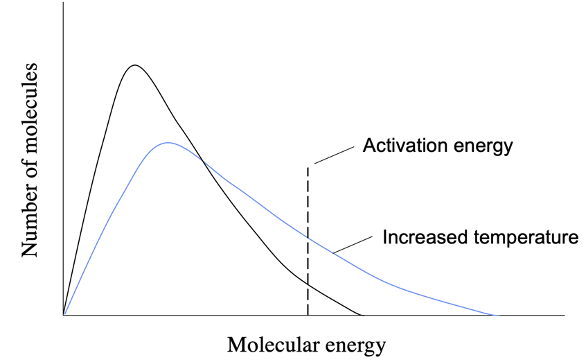The effect of increased temperature on molecular energy distribution

• Catalysts reduce the activation energy of a reaction. This does not affect the energy distribution of molecules as it does not change the energy inside the system. However, by reducing the activation energy, more molecules have enough energy to result in a chemical reaction when collisions occur.The effect of catalyst addition on molecular energy distribution

• In both cases, the frequency of a successful collision (one that results in a chemical reaction) increases, and so does the reaction rate.

### Collision Theory in Dynamic Equilibrium

• Chemical equilibrium is concerned with the reversibility of chemical reactions. Many reactions do not go to completion and instead both reactants and products exist in a dynamic equilibrium.
• An example of a reversible reaction that can exist in equilibrium is the reaction between N2O4 and NO2. Suppose a closed system initially has some amount of N2O4 and no NO2. Molecules of N2O4 can decompose to produce twice as many NO2.

$$2NO_{2(g)} \rightleftharpoons N_2O_{4(g)}$$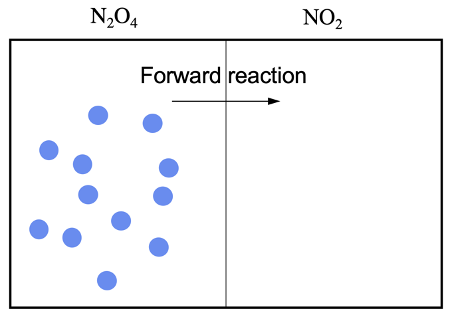• As the N2O4 molecules decompose to produce NO2, their concentration decreases and so does their collision rate. This in turn reduces the rate of the forward reaction.
• As NO2 molecules form, they can also collide and result in a reaction to re-form N2O4. This is represented by the reverse reaction.
• In the beginning, the forward reaction rate is greater than the reverse reaction rate as there are far more N2O4 than NO2 molecules. This results in a net decrease in [N2O4] and a net increase in [NO2]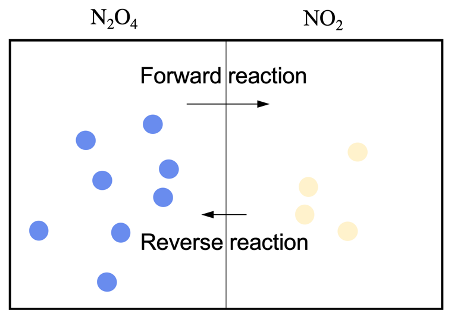• However, as the reaction proceeds, the rate of the forward reaction gradually decreases, and the rate of the reverse reaction gradually increases.• This continues until the rates of the forward and reverse reaction become equal, where equilibrium is established. At this point, forward and reverse reactions still occur due to the collision between molecules but no changes in concentration are observed.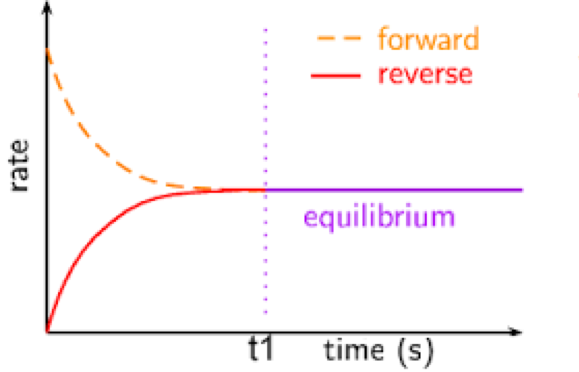Figure: change in reaction rate with time. At t1, rate of forward and backward reactions becomes equal, and equilibrium is reached.Figure: change in concentration of reactants and products of a reversible reaction.
[N_2O_4] decreases while [NO_2] increases. A reaction can reach equilibrium starting with any quantities of reactants and products.

## Practice Question

Using collision theory, explain the changes in [N2O4] and [NO2] shown by the graphs above.

• Initially, N2O4 collide and will result in a reaction to produce NO2 if the activation energy is met. This causes [NO2] to increase and [N2O4] to decrease.
• As [N2O4] falls, there are fewer collisions between N2O4 molecules, so the rate of the forward reaction decreases.
• As [NO2] increases, there are more collisions between NO2 molecules, so the rate of the reverse reaction increases.
• Forward and backward reaction rates will continue to change until they become equal in value. When this occurs, the reaction reaches chemical equilibrium. Since the two rates are equal, there is no change in [N2O4] and [NO2]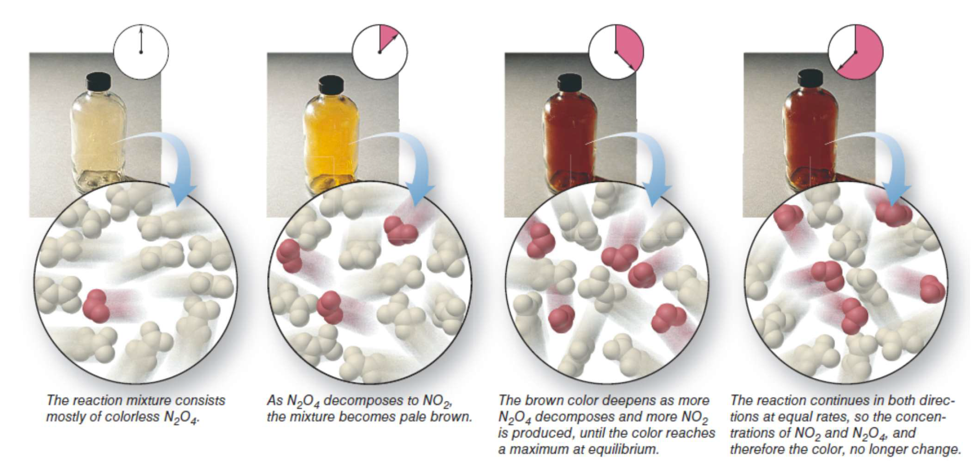Figure: Reaction between dinitrogen tetroxide and nitrogen dioxide can be monitored by its colour change. Nitrogen tetroxide is colourless whereas nitrogen dioxide has a distinctive brown colour.

• Nitrogen dioxide (NO_2) has a distinctive brown colour while dinitrogen tetroxide (N_2O_4) is colourless.
• In the reaction above, the reaction mixture is pale brown as it consists of mostly N_2O_4. As the reaction approaches equilibrium, the concentration of NO_2 increases, making the mixture appear browner.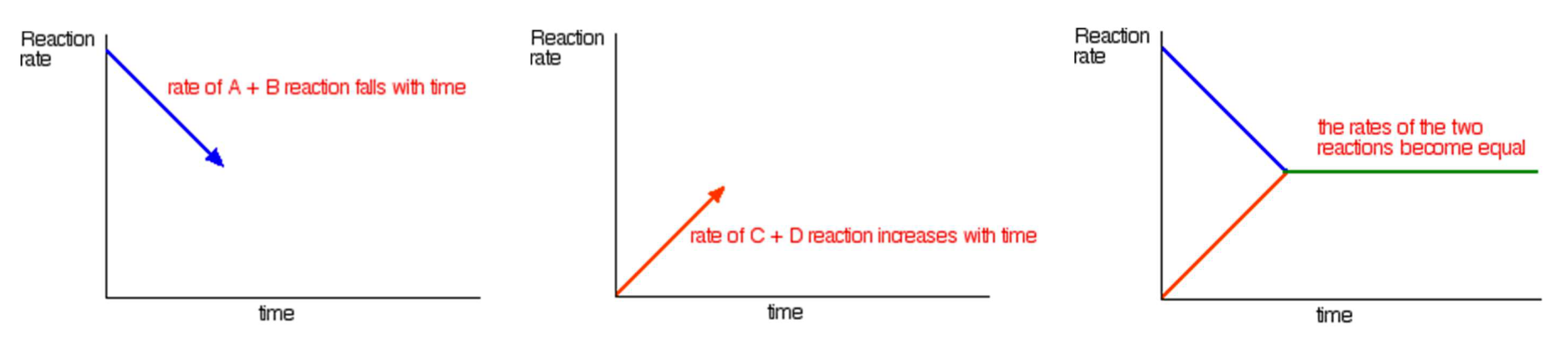Figure: Change in rates of forward and reverse reactions. In the beginning only reactants are present. Since there are no products, collision rate between products is zero, and so is the reverse reaction rate.

• As the concentration of N_2O_4 decreases, the collision rate of forward reaction decreases. On the other hand, as concentration of NO_2 increases, the collision rate of reverse reaction increases. These changes continue until they become equal, which marks when a dynamic equilibrium is achieved.

Previous section: Non-equilibrium Systems

Next section: